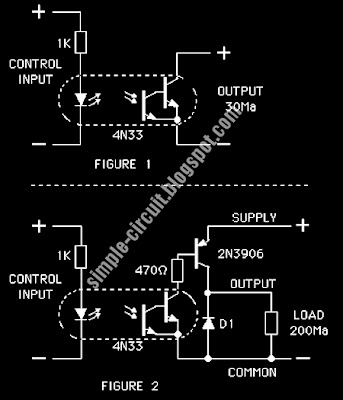Custom Search
WELCOME SIMPLE CIRCUIT DESIGN

## Wednesday, December 2, 2009

### Simple Basic Solid State Relays

This is the design for basic relays. This is a simplest form circuit. This is a solid state relays that could be used to replace mechanical types in many model railroad circuits. This is the figure of the circuit.This circuit is design to so that with a controlling current of 10 Milliamps flowing through the LED the voltage drop across the output will be less than 0.3 volts at the circuits rated current. The control and load supply voltages for the examples is 12 Volts, other voltages can be used with a corresponding adjustment to resistors in the circuit. For example if the voltages are doubled then the value of the 1K and/or 470 Ohm resistors should also be doubled in order to keep the currents through the LED and base of Q1 at the same level. If the 2N3906 transistor is replaced with Darlington type, larger currents can be handled, 1 Amp and more, but the voltage drop across the relay increases and the cost of each unit rises. The diode D1, at the output of FIGURE 2 is a 1N4001. This is an anti-ringing diode and is only really needed if there is an inductive load such as a solenoid being controlled.